# Surface Area and Volume Formulas

## Cube – Surface Area and Volume Formulas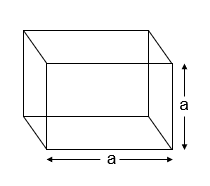Here,

a = side

Lateral Surface Area of a Cube –$4{ a }^{ 2 }$ sq units

Total Surface Area of a Cube –$6{ a }^{ 2 }$ sq units

Volume of a Cube –${ a }^{ 3 }$ cubic units

## Cuboid – Surface Area and Volume Formulas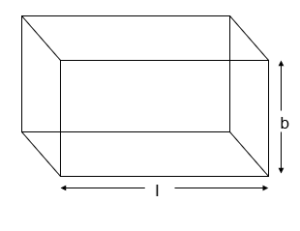Here,

l = length

b = breadth

h = height

Lateral Surface Area of a Cuboid – [2(l + b) x h] sq units

Total Surface Area of a Cuboid – 2(lb + bh + hl) sq units

Volume of a Cuboid – (l x b x h) cubic units

## Cylinder – Surface Area and Volume Formulas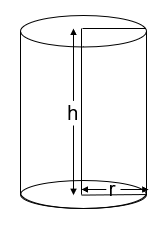Here,

r = radius

h = height

Curved Surface Area of a Cylinder –$2\pi { r }{ h}\quad sq\quad units$

Total Surface Area of a Cylinder –$2\pi { r }(h+r)\quad sq\quad units$

Volume of a Cylinder –$\pi { r }^{ 2 }h\quad cubic\quad units$

## Cone – Surface Area and Volume Formulas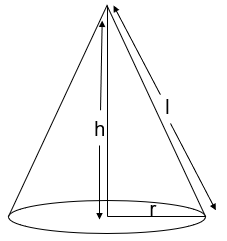Here,

h = height

r = radius

l = slant height

Curved Surface Area of a Cone –$\pi { r }{ l}\quad sq\quad units$

Total Surface Area of a Cone –$\pi { r }(l+r)\quad sq\quad units$

Volume of a Cone –$\cfrac { 1 }{ 3 } \pi { r }^{ 2 }h\quad cubic\quad units$

## Sphere – Surface Area and Volume Formulas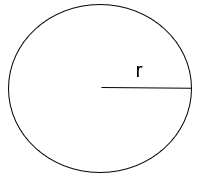Here, r = radius

Surface Area of a Sphere –$4\pi { r }^{ 2 }\quad sq\quad units$

Volume of a Sphere –$\cfrac { 4 }{ 3 } \pi { r }^{ 3 }\quad cubic\quad units$

## Hemisphere – Surface Area and Volume Formulas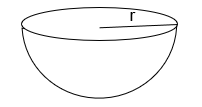Here, r = radius

Curved Surface Area of a Hemisphere –$2\pi { r }^{ 2 }\quad sq\quad units$

Total Surface Area of a Hemisphere –$3\pi { r }^{ 2 }\quad sq\quad units$

Volume of a Hemisphere –$\cfrac { 2 }{ 3 } \pi { r }^{ 3 }\quad cubic\quad units$